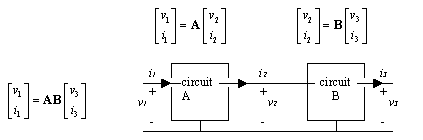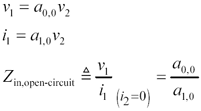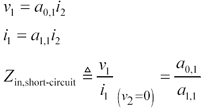# Appendix C. Two-Port Analysis

Figure C.1 depicts a generalized two-port circuit. The conventions for voltage and current preferred by this author are indicated. The figure assumes that both input and output ports share a common reference terminal, which is not strictly required for two-port analysis to work, but since the assumption conforms to the general situation in transmission-line analysis, the figure has been drawn that way.

Figure C.1. Any linear, time-invariant , two-port circuit can be represented by a four-element transmission matrix.Two-port circuits are much studied in the field of electrical engineering, and you will find many references dealing with them. Beware that there is a great variation in the conventions for polarity of input and output currents and voltages, and in the definition of the elements that comprise the four-element matrix used to describe the frequency-response properties of the circuit.

Underlying all of two-port analysis is the assumption that the circuit under study is both linear and time-invariant. This assumption renders possible the use of a frequency-based description of the circuit. Nonlinear circuits do not succumb to linear two-port analysis.

In the figure, the four elements of the transmission matrix A prescribe the actions of the circuit. Each of the four elements of A is itself a function of frequency . The matrix specifies, for any particular frequency, the allowed relationships between the input and output currents and voltages at that frequency .

Of all the possible two-port definitions, your author has selected the transmission matrix version of two-port analysis for this book because it simplifies certain calculations often performed for cascaded systems . To explain, suppose two systems represented by two-port matrices A and B are cascaded as shown in Figure C.2. Matrix B converts the output parameters ( v 3 , i 3 ) into ( v 2 , i 2 ), and then matrix A converts ( v 2 , i 2 ) into ( v 1 , i 1 ). The overall two-port description of the system spanning from ( v 1 , i 1 ) to ( v 3 , i 3 ) is therefore given by the matrix product AB . This simple method of chaining together the two-port matrices accounts for the popularity of the transmission-matrix formulation.

Figure C.2. A cascade of circuits may be represented by multiplying their transmission matrices.Let us derive from the four elements of a transmission matrix A the input impedance and gain of the circuit. The input impedance is calculated first assuming the output circuit ( v 2 , i 2 ) is open -circuited, meaning that i 2 = 0. Under that condition the matrix equations for ( v 1 , i 1 ) simplify to

Equation C.1What if the output of the circuit is shorted to ground? In that case v 2 = 0, and the input impedance is again calculated using simplified matrix equations:

Equation C.2In the case of an open-circuited output, the voltage transfer function is computed from the first line of [C.1].

Equation C.3High-Speed Signal Propagation[c] Advanced Black Magic
ISBN: 013084408X
EAN: N/A
Year: 2005
Pages: 163